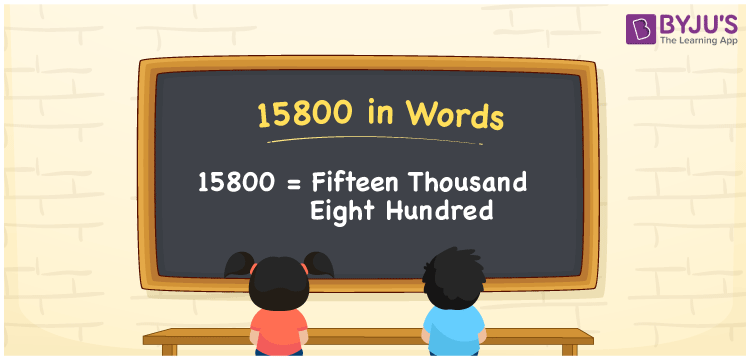# 15800 in Words

The number 15800 in words is “Fifteen thousand eight hundred”. In Mathematics, 15800 is a cardinal number that signifies the object’s value or the count. The place value table can be used to convert the number 15800 to words quickly and easily. For example, if the cost of the TV is Rs. 15800, it can be written as “The cost of the TV is Rs. Fifteen thousand eight hundred. In this article, we will learn how to write the number 15800 in words, as well as its conversion procedure.

 15800 in Words: Fifteen Thousand Eight Hundred. Fifteen Thousand Eight Hundred in Numerical Form: 15800.

## 15800 in English Words

The number 15800 in English words is written as follows:## How to Write 15800 in Words?

Using the place value system, the number 15800 can be written in words. Below is a table of place values for the number 15800.

 Ten-thousands Thousands Hundreds Tens Ones 1 5 8 0 0

We can expand the number 15800 as follows:

= 1 × Ten thousand + 5 × Thousand + 8 × Hundred + 0 × Ten + 0 × One

= 1 × 10000 + 5 × 1000 + 8 × 100

= 10000 + 5000 + 800

= 15800

= fifteen thousand eight hundred

Hence, 15800 in words is fifteen thousand eight hundred.

15800 in words – Fifteen thousand eight hundred

Is 15800 an odd number? – No

Is 15800 an even number? – Yes

Is 15800 a perfect square number? – No

Is 15800 a perfect cube number? – No

Is 15800 a prime number? – No

Is 15800 a composite number? – Yes

## Frequently Asked Questions on 15800 in Words

Q1

### How to write 15800 in words?

15800 in words is fifteen thousand eight hundred.

Q2

### Simplify 15000 + 800, and express in words.

Simplifying 15000 + 800, we get 15800. Hence, 15800 in words is fifteen thousand eight hundred.

Q3

### How to write fifteen thousand eight hundred in numbers?

Fifteen thousand eight hundred in numbers is 15800